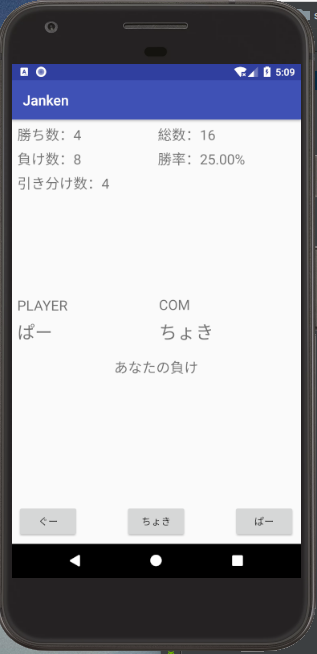# [JAVA] I made a rock-paper-scissors app with android

I added the win / loss count and the winning percentage to the basic rock-paper-scissors. Obviously, the more you do, the closer the winning percentage will be to 33.3%.#### `MainActivity.java`

``````
package to.msn.wings.janken;

import android.support.v7.app.AppCompatActivity;
import android.os.Bundle;
import android.view.View;
import android.widget.TextView;
import java.util.Random;

public class MainActivity extends AppCompatActivity {

/*
TextView:
player(Player text),playerRes (player's hand)
com(Com text), comRes(Com hand)
result (win / loss result)
winCnt (win rate),loseCnt,drawCnt (number of draws)
totalCnt,winPer (win rate)
button:
gu, tyoki, pa
*/

private int totalCnt; //Total number of battles
private int drawCnt; //Number of draws
private int loseCnt; //Number of losses
private int winCnt; //Number of wins

@Override
protected void onCreate(Bundle savedInstanceState) {
super.onCreate(savedInstanceState);
setContentView(R.layout.activity_main);
}

public void btn_onclick(View view){

//Hand on the player side
TextView txt = (TextView)findViewById(R.id.playerRes);
int player_hand = 0;
//view.Judge the button clicked with getId
// 0:gu, 1:tyoki, 2:par
switch (view.getId()){
case R.id.gu:
txt.setText("Goo");
player_hand = 0;
break;
case R.id.tyoki:
txt.setText("Choki");
player_hand = 1;
break;
case R.id.pa:
txt.setText("Par");
player_hand = 2;
}

//hand on the com side
TextView com = (TextView)findViewById(R.id.comRes);

//Set hands of com with random
Random random = new Random();
int n = random.nextInt(3);
int com_hand = 0;
// 0:gu, 1:tyoki, 2:par
if (n == 0){
com.setText("Goo");
com_hand = n;
} else if (n == 1){
com.setText("Choki");
com_hand = n;
} else if (n == ２){
com.setText("Par");
com_hand = n;
}

//Count up the total number of matches
TextView total = (TextView) findViewById(R.id.totalCnt);
totalCnt++;
total.setText("Total number:" + String.valueOf(totalCnt));

//Rock-paper-scissors win / loss judgment
TextView result = (TextView) findViewById(R.id.result);
int judge = (player_hand - com_hand + 3)%3;
if (judge == 0) {
//In this case
TextView draw = (TextView) findViewById(R.id.drawCnt);
drawCnt++;
draw.setText("Number of draws:" + String.valueOf(drawCnt));
result.setText("Aiko");
} else if (judge == 1) {
//If you lose
TextView lose = (TextView) findViewById(R.id.loseCnt);
loseCnt++;
lose.setText("Number of losses:" + String.valueOf(loseCnt));
} else if (judge == 2){
//If you win
TextView win = (TextView) findViewById(R.id.winCnt);
winCnt++;
win.setText("Number of wins:" + String.valueOf(winCnt));